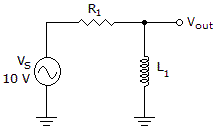# Electronics - RL Circuits - Discussion

### Discussion :: RL Circuits - General Questions (Q.No.11)

11.Which of the following statements is true if R = 100and XL = 100in the circuit in the given circuit?

 [A]. Each component drops 5 V. [B]. The impedance equals 200. [C]. The power factor equals 1. [D]. The phase angle equals 45°.

Explanation:

No answer description available for this question.

 Chandan said: (Aug 20, 2012) theta=tan-(xl/r) that is tan-(100/100) i.e is phase angle is equal to 45degree